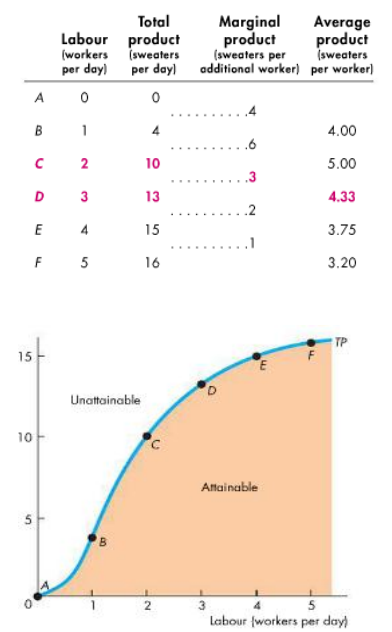# Economics 1021A/B Lecture Notes - Diminishing Returns, Average Variable Cost, Marginal Product

32 views6 pages
School
Department
Page:
of 6Chapter 11 Notes
Decision Time Frames
the goal of all firms is to maximize attainable profit
decisions are either made in the long run or the short run
short run
o time frame in which the quantity of at least one factor of production is fixed
typically capital, land, and entrepreneurship constant, labour variable
fixed factors of product called plant
in the short run, a firm’s plant is fixed
o short run decisions are easily reversed
the firm can change its output in the short run by changing the quantity
of labour it employees
long run
o time frame in which the quantity of all factors of production can be varied
o to increase an output in the long run, a firm can change its plant as well as the
quantity of labour it hires
o decisions not easily reversed
past expenditure on plant that has no resale value is referred to as a
sunk cost
Short Run Technology Constraint
total product is the total output of a good
produced
marginal product of labour is the increase
in total product that results from a one-unit
increase in the quantity of labour employed,
with all other input remaining the same
average product of labour is equal to total
product divided by the quantity of labour
employed
Total Product Curve
the total product curve shows quantities of
product that are attainable and unattainable
only points on the total product curve are
technologically efficient
Marginal Product Curve
the height of the curve measures the slope of
the total product curve
the total product and marginal product curves
differ across firms and types of goods
the shapes of product curves are similar due
to two features:
o increasing marginal returns initially
o diminishing marginal returns
eventually
increasing marginal returns
o occurs when the marginal product of
an additional worker exceeds the marginal product of the previous worker
o increasing marginal returns arise from increased specialization and division of
labour in the production process
diminishing marginal returns
o occurs when the marginal product of an additional worker is less than the
marginal product of the previous worker
o arise from the fact that more and more workers are using the came capital and
working in the same space
o as more workers are added, there is less and less for the additional workers to
do that is productive
o this phenomenon is called the law of diminishing returns
as a firm uses more of a variable factor of production with a given
quantity of the fixed factor of production, the marginal product of
the variable factor eventually diminishes
Average Product Curve
average product of labour shows the relationship
between average product and marginal product
average product is largest when it is equal to
marginal product
o the marginal product curbe cuts the average
product curbe at the point of maximum
average product
for the number of workers at which marginal
product exceeds average product, average product
is increasing
for the number of workers at which marginal product is less than average product,
average product is decreasing
Short-Run Cost
to produce more output in the short run, a firm must employ more labour, which
means it must increase its costs
o the relationship between output and cost is described by TOTAL,
MARGINAL, and AVERAGE COST
total cost
o the cost of all factors of production
and its uses
o separated into fixed and variable
costs
o total fixed cost is the cost of the
firm’s fixed factors, which does not
change with output
o total variable cost is the cost of the
firm’s variable factors (typically
labour), and changes as output
change
o TC = TFC + TVC
marginal cost
o the increase in total cost that results from a one-unit increase in output
o MC = ∆TC ÷ ∆Q
o at small outputs, marginal cost decreases as output decreases because of
greater specialization and the division of labour
o as output increases, marginal cost increases because of the law of diminishing
returns
output produced by each additional worker is successively smaller
o to produce an additional unit of output, more workers are required, and the
marginal cost must eventually increase
average cost
o average total cost is the total cost per unit of output
o average fixed cost is total fixed cost per unit of output
o average variable cost is total variable cost per unit of output
  



   
Marginal Cost and Average Cost
the marginal cost curve (MC) intersects the average variable cost curbe and the
average total cost curve at their minimum points
o when marginal cost is less than average cost, average cost is decreasing
o when marginal cost is greater than average cost, average cost is increasing
true for both average total cost and average variable cost
average total cost is the sum of average fixed cost, so the shape of the ATC curve
combines the shapes of the AFC and AVC curves
o U-shape arises from two factors
spreading fixed cost over a larger output
eventually diminishing returns
o when output increases, the firm spreads its total fixed cost over a larger
output, thus average fixed cost decreases